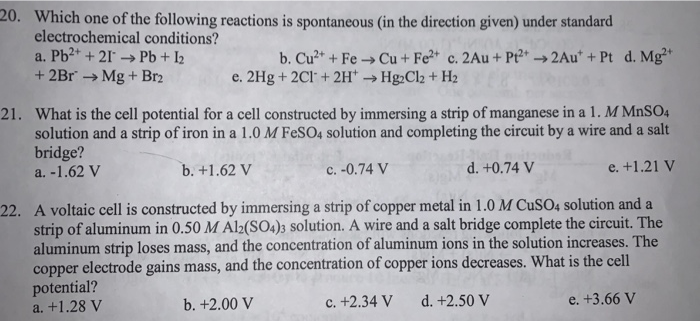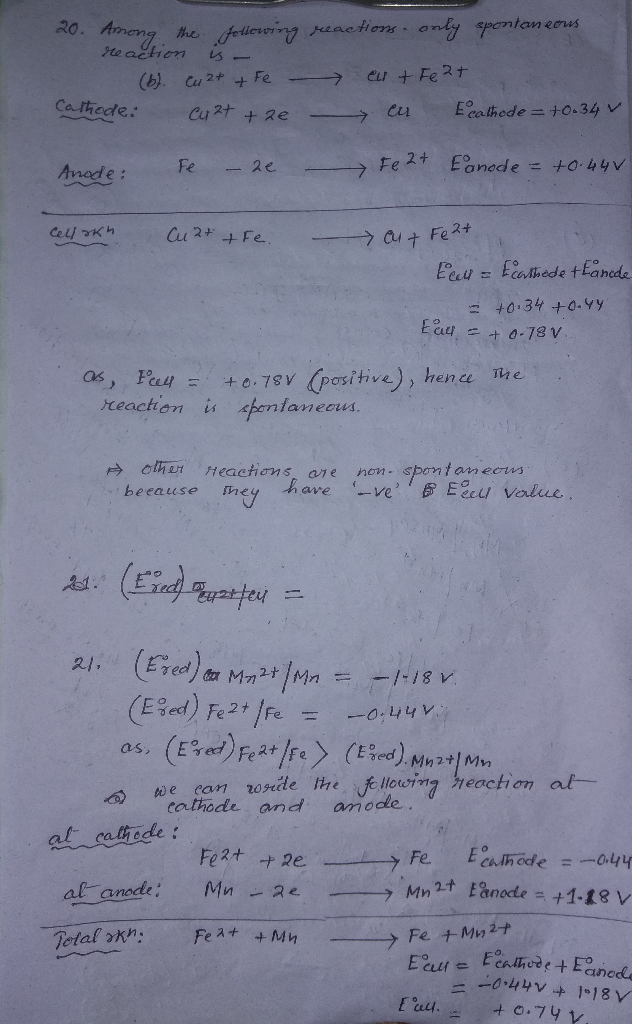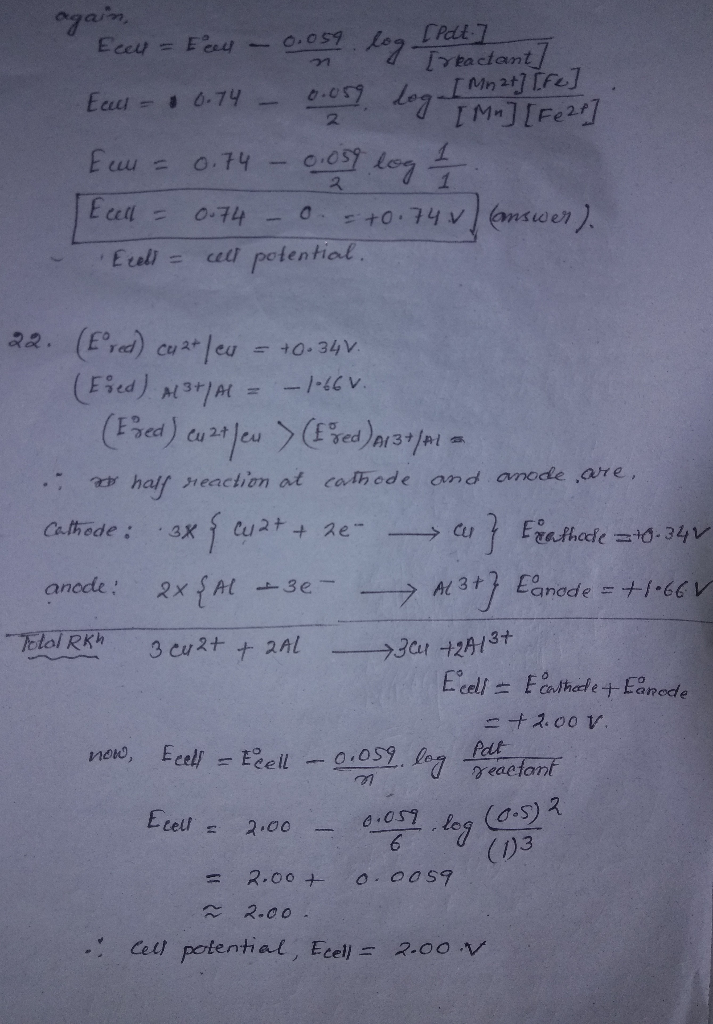Homework Help Question & Answers

# 20. Which one of the following reactions is spontaneous (in the direction given) under standard e...

Please answer the following questions!20. Which one of the following reactions is spontaneous (in the direction given) under standard electrochemical conditions? +2Br- Mg+ Br2 What is the cell potential for a cell constructed by immersing a strip of manganese in a 1. M MnSO4 solution and a strip of iron in a 1.0 M FesO4 solution and completing the circuit by a wire and a salt bridge? 21. b. +1.62 V c. -0.74 V d. +0.74 V e. +1.21 v A voltaic cell is constructed by immersing a strip of copper metal in 1.0 M CuSO4 solution and a strip of aluminum in 0.50 M Al2(SO4)3 solution. A wire and a salt bridge complete the circuit. The aluminum strip loses mass, and the concentration of aluminum ions in the solution increases. The copper electrode gains mass, and the concentration of copper ions decreases. What is the cell potential? a. +1.28 V 22. b. +2.00 V c. +2.34 V d. +2.50 V e. +3.66 V

#### Homework Answers

ReportAnswer #1Add Homework Help Answer
##### Add Answer of: 20. Which one of the following reactions is spontaneous (in the direction given) under standard e...
Your Answer: Your Name: What's your source?
Not the answer you're looking for? Ask your own homework help question. Our experts will answer your question WITHIN MINUTES for Free.
More Homework Help Questions Additional questions in this topic.

• #### Reactions of propene: Which can be made in one step from propene followed by acid work-up where appropriate? Please help. Reactions of Propene a. Which can be made in one step from propene CH3-CH CH2...

Need Online Homework Help?

Get FREE EXPERT Answers
WITHIN MINUTES
Related Questions